# 【论文阅读】Efficient Graph-Based Image Segmentation

## 图像分割，最小生成树（Minimum Spanning Tree，MST），kruskal算法，prim算法

Posted by x-jeff on October 19, 2021

# 1.Introduction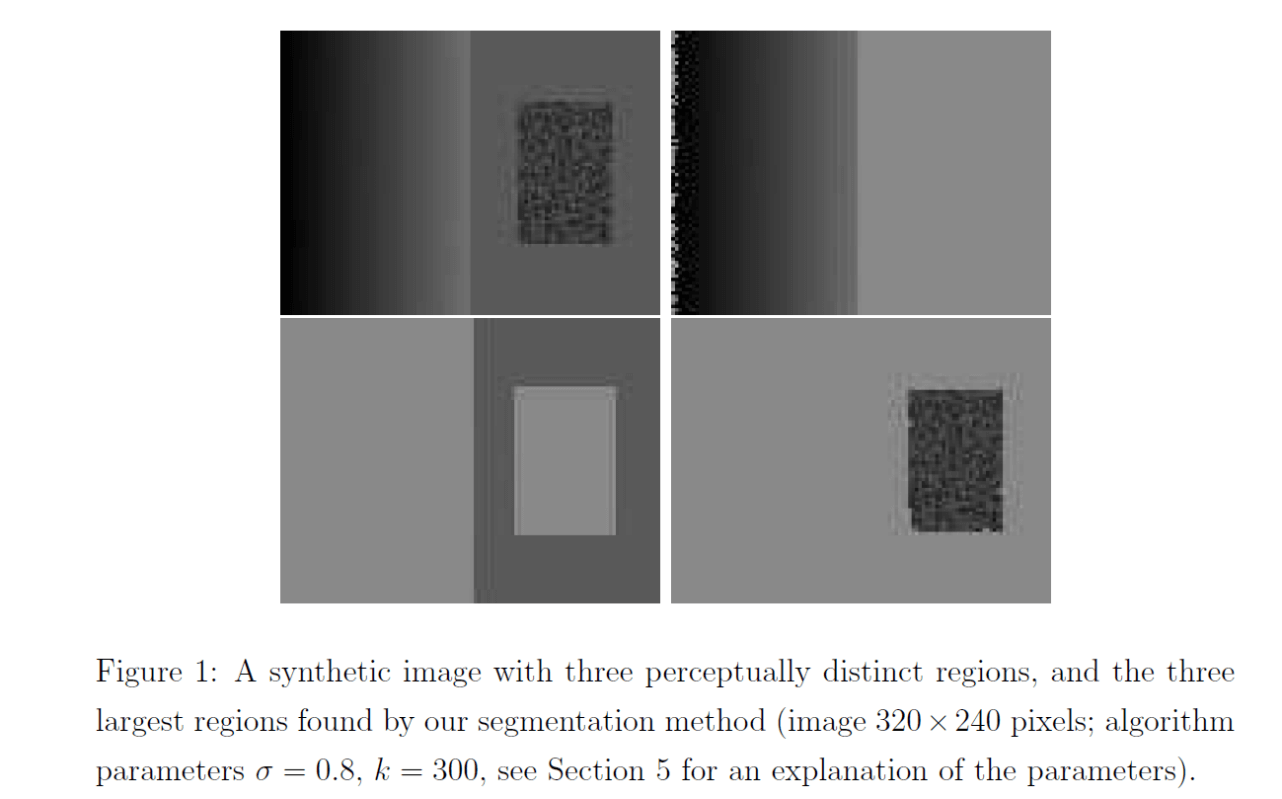Fig1剩余的三幅图像为我们算法分割的结果。

# 2.Related Work

MST是指保证所有节点连通且权值最小的树型结构。MST可以用kruskal算法（见本文第8部分）或prim算法（见本文第9部分）求出。

# 3.Graph-Based Segmentation

$G=(V,E)$为无向图，节点$v_i \in V$，边$(v_i,v_j) \in E$（$v_i,v_j$为相邻节点），边的权重$w((v_i,v_j))$（非负）。

## 3.1.Pairwise Region Comparison Predicate

$Int(C)=\max \limits_{e \in MST(C,E)} w(e) \tag{1}$

$Dif(C_1,C_2)=\min \limits_{v_i \in C_1,v_j \in C_2,(v_i,v_j)\in E} w((v_i,v_j)) \tag{2}$

$D(C_1,C_2) = \begin{cases} true, & if \ Dif(C_1,C_2) > MInt(C_1,C_2) \\ false, & otherwise \end{cases} \tag{3}$

$MInt(C_1,C_2) = \min (Int(C_1)+\tau (C_1),Int(C_2)+\tau (C_2)) \tag{4}$

$\tau (C)=k/\mid C \mid \tag{5}$

k是一个超参数。k越大，最终分割出来的区域越少，每个区域的面积就会越大。

# 4.The Algorithm and Its Properties

👉第零步：将边按其权重进行升序排列，得到$\pi=(o_1,…,o_m)$。

👉第一步：将每一个节点视为一个单独的区域，将其作为算法的初始分割$S^0$。

👉第二步：设$q=1,…,m$，循环第三步。

👉第三步：假设第q条边为$o_q=(v_i,v_j)$（$v_i,v_j$为该边所连接的两个相邻像素点），节点$v_i$属于区域$C_i^{q-1}$，节点$v_j$属于区域$C_j^{q-1}$，区域$C_i^{q-1}$和区域$C_j^{q-1}$都属于分割$S^{q-1}$。如果$C_i^{q-1} \neq C_j^{q-1}$且$w(o_q) \leqslant M Int (C_i^{q-1},C_j^{q-1})$，则合并区域$C_i^{q-1}$和区域$C_j^{q-1}$，否则不合并（即$S^q=S^{q-1}$）。

👉第四步：遍历完所有的边后，得到最后的分割结果$S^m$。

# 5.Results for Grid Graphs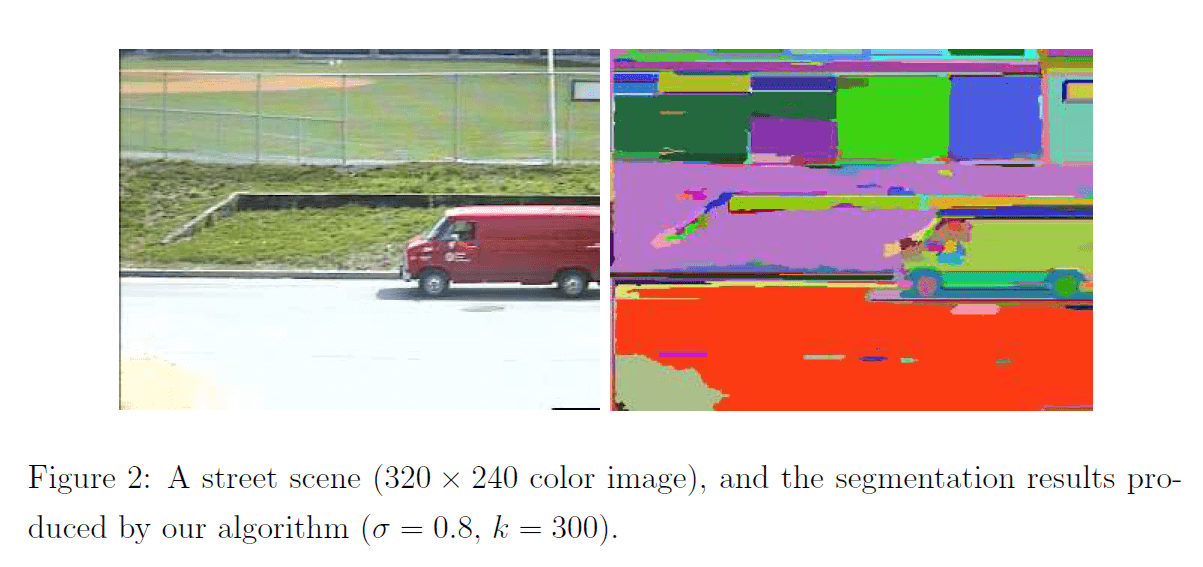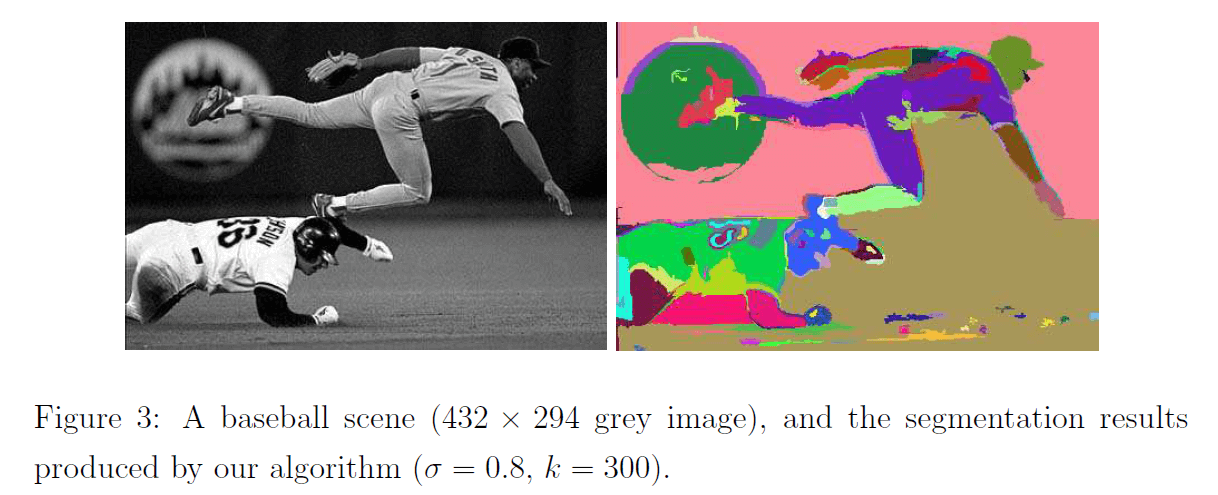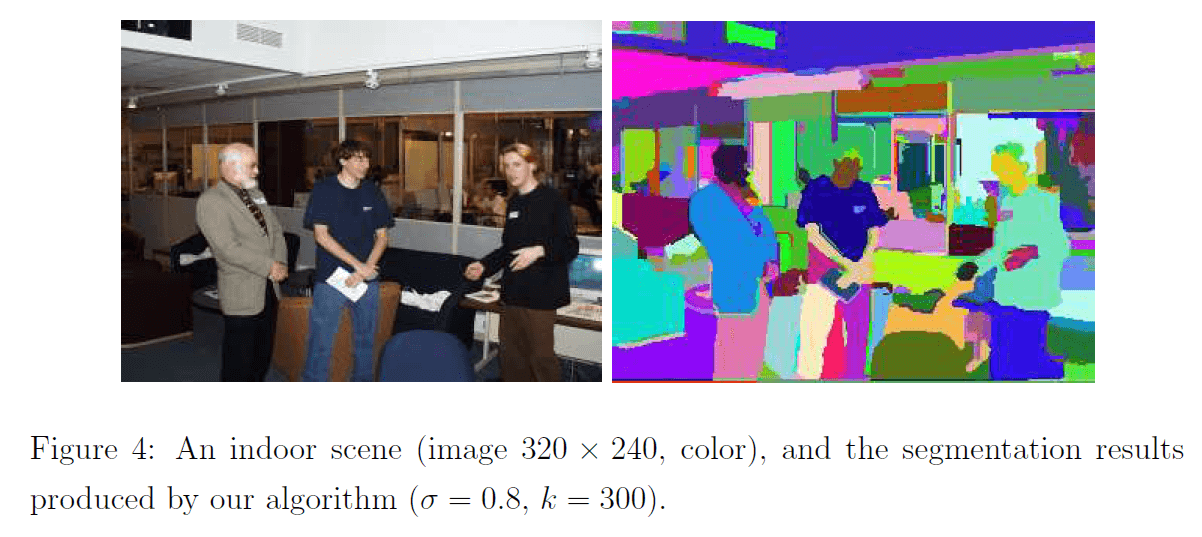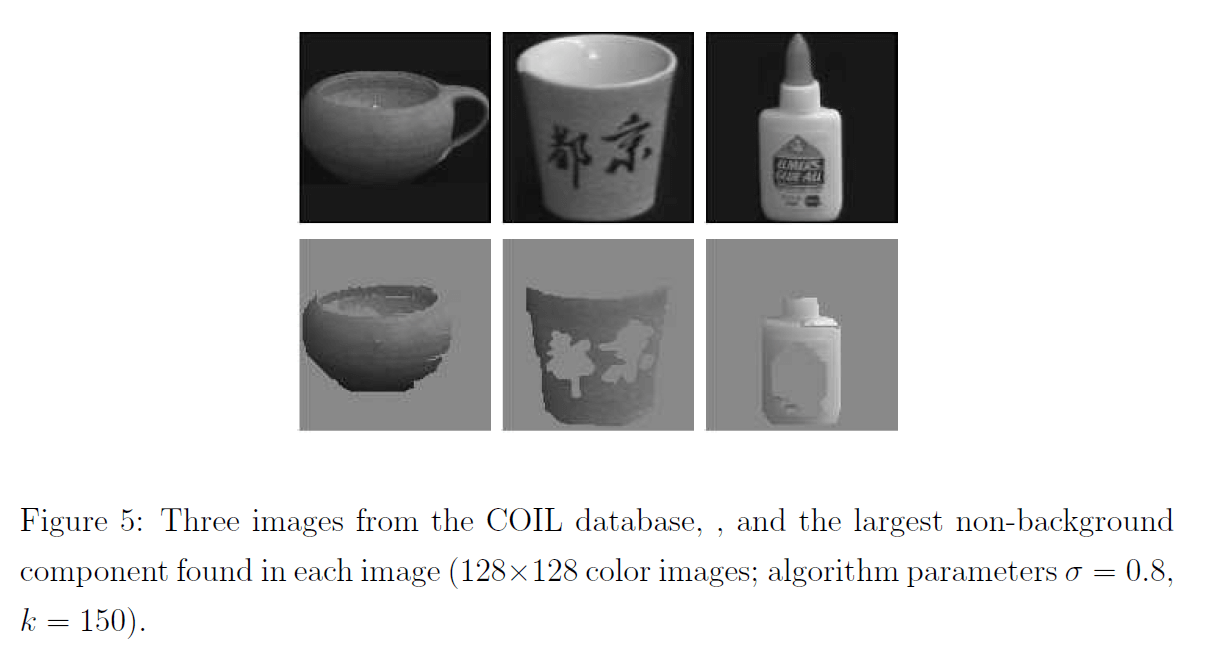# 8.kruskal算法

1. 将图中的所有边都去掉。
2. 将边按权值从小到大的顺序添加到图中，保证添加的过程中不会形成环。
3. 重复上一步直到连接所有顶点，此时就生成了最小生成树。这是一种贪心策略。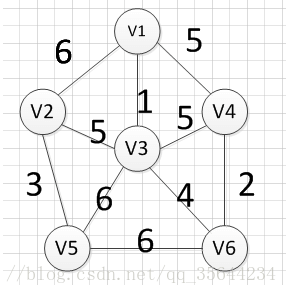MST的生成步骤见下：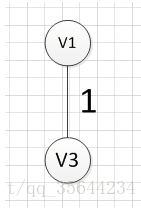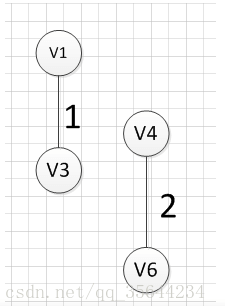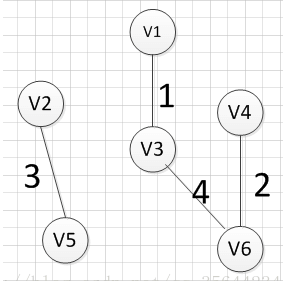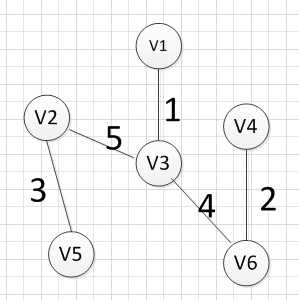# 9.prim算法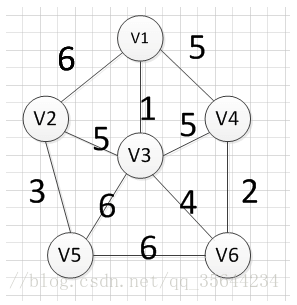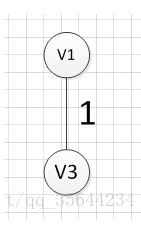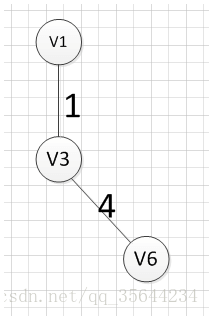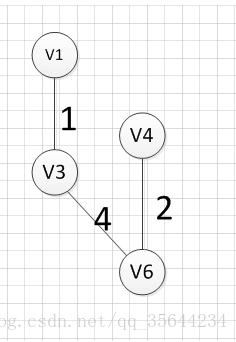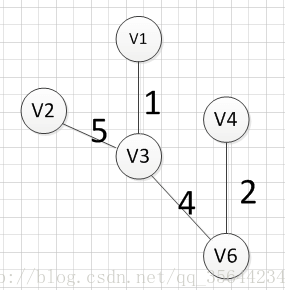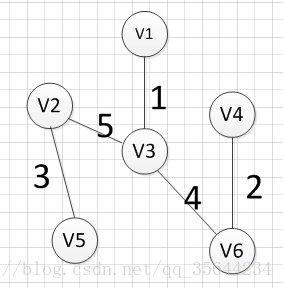# 11.参考资料